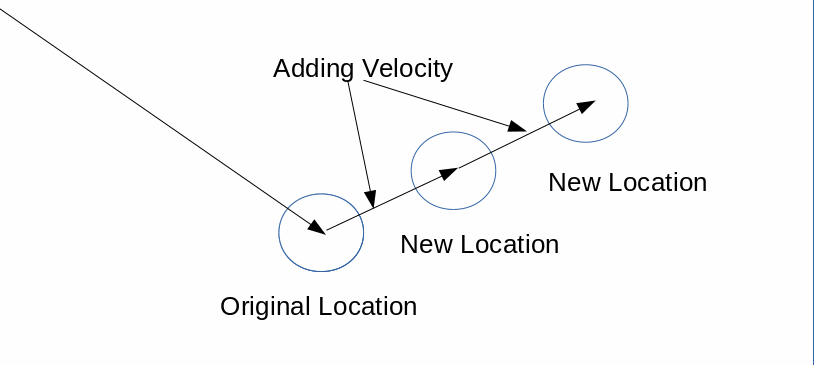28 February 2018, 18:12

# acceleration

This year has really got off to a flying start. I'm super busy at work and school and I have been squeezing in non work related learning when I can. I have had to do more database work at my day job so I have been wrapping my head around that which has stunted my progress with the Nature of Code.

That's not going to stop me getting to it when I can, It just my progress will be a little slower.

I managed to get some time this morning to take some notes.

## Acceleration

Let's recap quickly, before we were looking at this example:

This is a ball that bounces around the screen. If we look at the code, we can see that the ball object has two vectors, location ( where it is ) and velocity ( change in location over time ).

In our update function we add velocity to location. This gives the ball movement.

Instinctively to make this ball move differently you may think it would be a good idea to change velocities x and mess around with location, add random to location y. All these things directly mess with location and velocity.

Let's assume that this is not a good idea, A cleaner approach would be to add another vector that represents a force acting on the ball. Let's look at what is happening here:In the diagram we are looking at a snapshot of movement. We can see the impact that adding velocity to location has over time. If we wanted to make the velocity change we could potential mess around with the internal variables of the vector. This may work now, but as things get more complex it will gradually get harder to keep track of all the variables.

Instead we could add another vector, In this case acceleration. Just as velocity effects location over time, acceleration effects velocity over time.

Acceleration is any change in velocity over time, it could be the magnitude ( size ) of the velocity ( speed ) the direction of the velocity.

Let's add acceleration to our original bouncing ball:

### Constant Acceleration

In this example the acceleration vector is slowly added to the ball over time, you can see that the ball will move faster and faster as the acceleration accumulates.See the Pen Simple Bouncing Ball Vector With Constant Acceleration by Adam Harpur (@harps116) on CodePen.

change the value of the acceleration vector's x value to see the effect on the balls velocity. You will notice that things will get very fast if the value is over 0.5. This is because every frame the acceleration is increasing by that x value. We are running our animation at the default p5 rate of 60 frames per second. So hopefully you can see how things get very fast in a short span of time.

### Constant acceleration with velocity

In this example we are adding acceleration to an existing velocity that is moving two pixels downward.See the Pen Simple Bouncing Ball Vector With Constant Acceleration by Adam Harpur (@harps116) on CodePen.

with this approach is almost like a wind is acting on the ball that is getting stronger and stronger. The acceleration vectors x component is very low in order to really see the full effect.

### Random acceleration.

Here we use the handy p5 Vector function 'vector_instance'.random2D() which gives us a random vector with a random x and y component. Note that we wouldn't want a z component as we are still working in 2D.See the Pen Random Acceleration and Velocity by Adam Harpur (@harps116) on CodePen.

Check out Daniel Shiffman's great series on the Nature of Code, this 100 days of learning is a great excuse for me to finally work through the lessons and take some notes as I go.

← Force
Vectors →